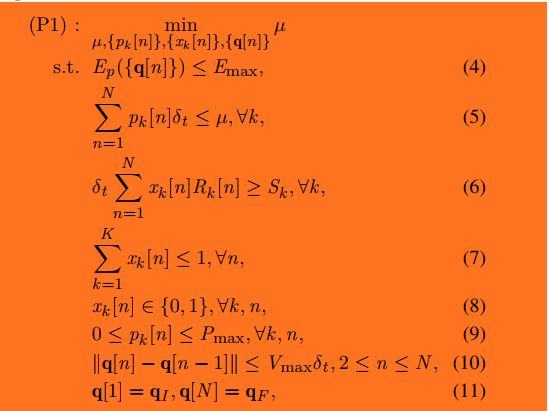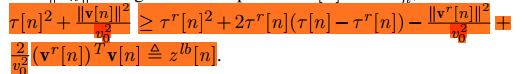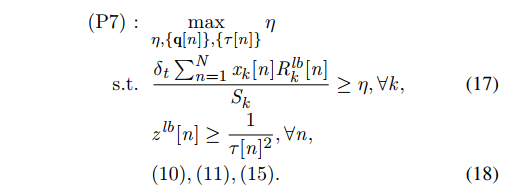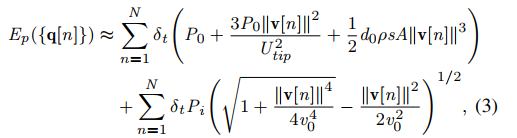# Disciplined convex programming error: Cannot perform the operation: {convex} >={convex}

Hi,everyone.I have a question about the CVX.
The following constraints are known to be convex：
`2*A*X-A^2+2*B*Y-B^2>=1./X^2.`
（Among them, X and Y are both variables）
However,when I wrote this constraint in the CVX as above,the MATLAB showed that:
error - cvxprob/newcnstr (line 192)
Disciplined convex programming error:
Invalid constraint: {convex} >= {convex}.
I want to know how can I do to make CVX run normally.
Thank you all.

I don’t see how the LHS would be convex, unless `A` or `B` were suitable CVX expressions, such as `A = X` or `B = Y`.

Please show a complete reproducible problem with all input data provided, all variables declared, etc, along with the output.

My question comes from this paper:
https://ieeexplore.ieee.org/document/8714077
The original problem isThe figure above is the description of the trajectory sub-problem，among this, tau[n] is a slack variable to transform the non-convex subproblem to a convex problem. By using the first-order Taylor expansion，this paper gets the convex constraint,like this:(16)
Here,the question becomes a convex problem and can be solved by CVX ,like this:But I don’t know how to express this problem in CVX,can you help me ,Mark?

If `v`, and hence `z`, is input data, how did you come up with that crazy LHS in your original question, and why would CVX determine that LHS to be convex? I am not sure, because I haven’t actually seen the paper, but from what you show, it appears that problem P7 consists of the objective, 17, 18, 10, 11,15 (I don’t know what 15 is). It appears (but I’m not sure it’s the case) that the inequality constraint on the first line of 16 is not part of the P7 formulation, and therefore should not be entered into CVX. I suggest you read the paper carefully to determine exactly what needs to go into the CVX problem formulation.

I recommend starting again, and do things carefully. You have not provided a combination of code and error message which would have gone together.

16 is defined as z_lb[n]
constraint 15 is here15:Ep({q[n]}) <=Emax

At this point, what you need to do is

1. understand the paper

2. understand exactly what the optimization problem you wish to enter in CVX is, including all definitions, notation, and conventions

3. understand why it is a convex optimization problem

Until you have done that, you are not ready to use CVX on the problem.

thanks, Mark.
i think i should read this paper carefully